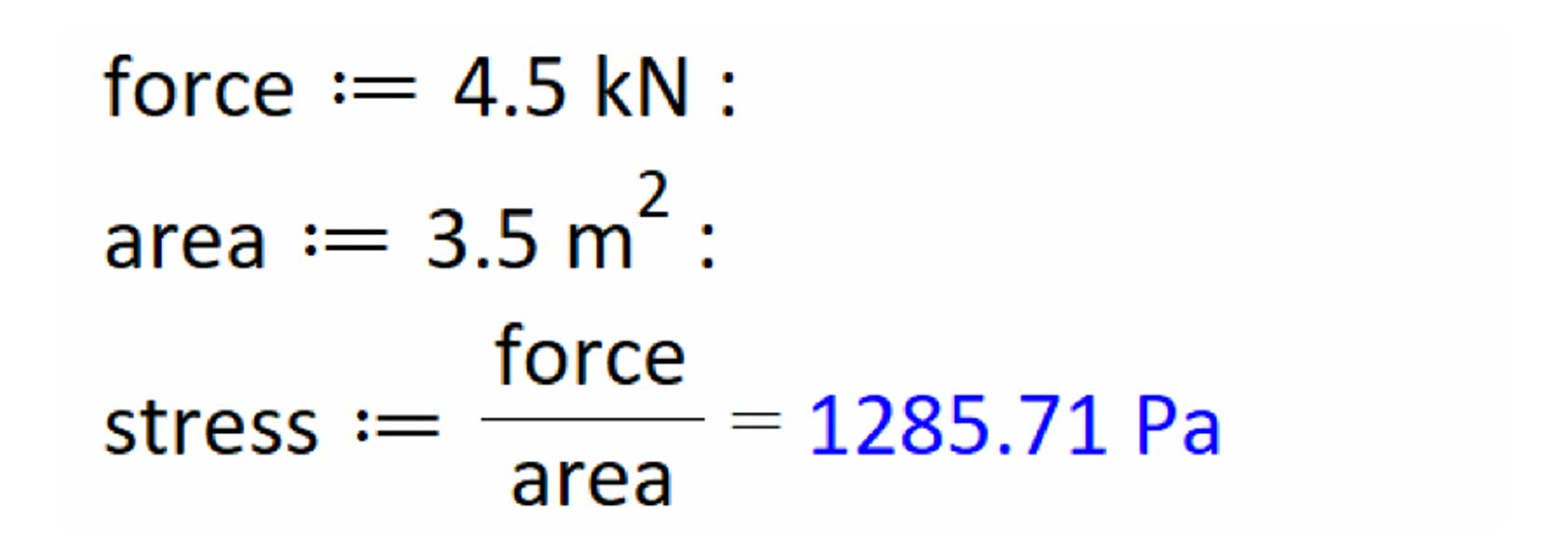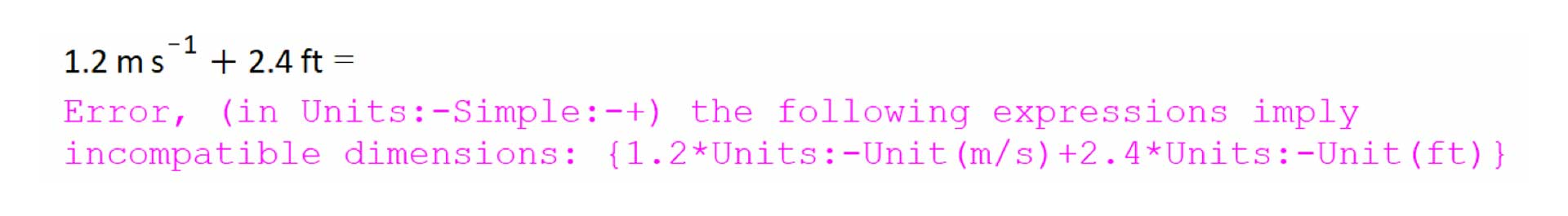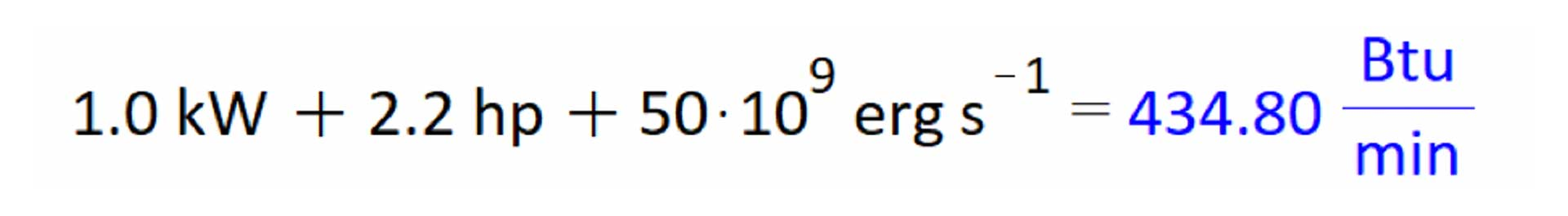Reduce Calculation Risk with Units-Aware Math Software - Maplesoft

# Reduce Calculation Risk with Units-Aware Math Software

## Abstract:

Nearly every quantity an engineer deals with has a unit. In fact, dimensionality is a fundamental characteristic of engineering calculations.

However, most calculation software tools do not understand the concept of dimensionality; unit conversions and consistency checks need to be done manually.

In this whitepaper, we will demonstrate how Maple is a units-aware calculation tool that solves problems with units that engineers encounter in other software.

Video: Learn how calculation management can play a central role in organizing your work, and see examples from various engineering domains. Learn more about Maple & Calculation Management

This is especially onerous with spreadsheets, where:

• Conversion factors randomly appear in a formula (either in a cell on its own, or embedded in a formula). This reduces the readability and auditability of calculations.
• These conversion factors are often not documented, leaving you perplexed and confused.

In the worst cases, unit conversion errors can lead to engineering disasters. For example, in 1999, NASA lost a \$125 million space probe because one team worked in metric while another worked in US Customary units. While your unit conversion errors may not be as disastrous as those suffered by NASA, they're still frustrating, time consuming and a source of risk. And every engineer has an ethical duty to reduce risk.Figure 1: Spreadsheets have unit conversion factors appearing out of nowhere

Simply by using units-aware calculation software throughout a technical analysis, you eliminate unit conversion errors and provide a check on the physical validity of your equations. This saves time, removes a source of frustration and increases the reliability of your analyses.

Before you start a project, make sure your calculation tool understands units. Here's what to look for.

## Readability and Clarity of Equations and Units

Equations should be presented naturally, and in a form that requires little cognitive overhead to understand.Figure 2: Readability and clarity of units

## Automatic Unit Conversion

You should be able to add or subtract parameters with the same fundamental dimensions, with all unit conversion done automatically.Figure 3: Automatic unit conversion

Results should be easily converted into different units, preferably with as few mouse clicks or keystrokes as necessary.Figure 4: Reconciliation of units

## Reconciliation of Units

Compound units should, when appropriate, be automatically reconciled into simpler units. For example:

• N/m2 should be converted to Pa
• A current multiplied by a voltage should usually be converted to watts.Figure 5: Reconciliation of units

## Adding Dimensionally-Inconsistent Units Produces Errors

Your calculation software should tell you if you're trying to add quantities that don't have the same units, and refuse to proceed until you've corrected the error.Figure 6: Unit errors should be flagged

This is a simple check to ensure your equations are physically valid.

## Wide Range of Units and Unit Systems

At the very minimum, your calculation tool should understand the entire range of SI and US Customary units (with, of course, a smooth flow of calculations between both unit systems).

Some niches in chemistry and physics use the CGS or Atomic unit system; these should preferably be supported as well.Figure 7: Broad range of units and unit systems

## Units in Solvers, Integrators and Optimizers

Engineering systems often result in sets of equations that demand numerical solvers, integrators or optimizers. For example,

• Stub matching involves calculating the distance of a segment of a transmission line from a complex load; the resulting equations need to be solved numerically
• Calculating the work done in the isothermal compression of a gas involves numerically integrating pressure over the change in specific volume.
• Mechanical optimization involves minimizing the cost or weight of a design while maintaining constraints on viable dimensions or deformations.

Accordingly, these computational routines should understand units.Figure 8 (Left): Units in numerical solution of equations     Figure 9 (Top-Right): Units in numerical integration     Figure 10 (Right): Units in numerical optimization

## Engineering and Scientific Data Should Use Units

Engineers and scientists often employ libraries of data in calculations. Some examples include:

• Fluid properties, like enthalpies and viscosities
• Chemical properties, like molecular weights
• Scientific constants, like the Boltzmann constant
• Structural properties, like the properties of standard steel shapes

Any collections of scientific or engineering data built into your tool should include, wherever appropriate, unit information.Figure 11: Units associated with scientific and engineering data

## Plotting and Visualization

Units should be supported in plots and visualization routines, with dimensions clearly indicated on axes labels.Figure 12: Units in plots

## Conclusion

Calculation software tools already provide high-level tools for math, programming and visualization. Very few of these tools, however, understand units.

A lack of units in your calculation tool can introduce a source of risk into your technical analyses; the probability of unit-conversion errors increases rapidly when analyses are shared within and between teams.

Maple is a units-aware calculation tool, and was used to produce all the examples in this article. With Maple, units can be associated with parameters, carried through an analysis, and used in numerical solving and optimization. By using Maple, engineers eliminate unit conversion errors, saving time and increasing the reliability of their analyses.

## Bereit für den nächsten Schritt?

*Die Maple-Evaluation ist für Schüler und Studenten bzw. die private Nutzung zurzeit nicht verfügbar.